# Electronics and Communication Engineering - Automatic Control Systems

### Exercise :: Automatic Control Systems - Section 2

46.

A system has transfer function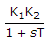. If a sinusoidal input of frequency ω is applied the steady state output is of the form

 A.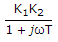B. K1 K2 (1 + jω/T) C. K1K2/jωT D.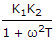Explanation:

For sinusoidal input jω replaces s in the transfer function.

47.

For the given figure C(s)/R(s) =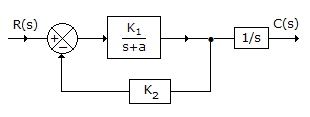A.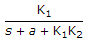B.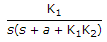C.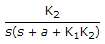D.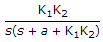Explanation: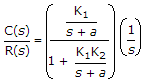or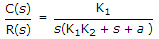.

48.

For a quadratic factor in transfer function the phase angle is not a function of damping factor.

 A. True B. False

Explanation:

Damping ratio affects phase angle.

49.

The current rating of dc tachogenerator is usually

 A. small B. high C. medium D. either (b) or (c)

Explanation:

Control systems require small current rating of components.

50.

For the compensator in the given figure the maximum phase lead is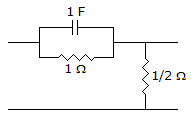A. sin-1 (1/3) B. 30° C. 45° D. 60°

Explanation: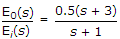Hence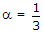Also sin φm =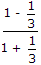= 0.5 and φm = 30°.